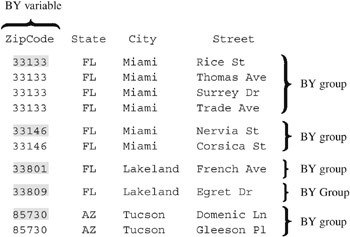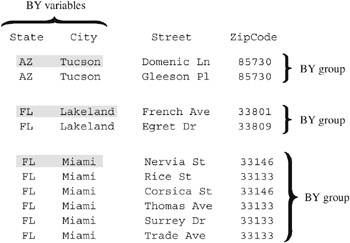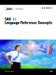# Understanding BY Groups

### BY Groups with a Single BY Variable

The following figure represents the results of processing your data with the single BY variable ZipCode. The input SAS data set contains street names , cities, states, and ZIP codes that are arranged in an order that you can use with the following BY statement:

` by ZipCode; `

The figure shows five BY groups each containing the BY variable ZipCode. The data set is shown with the BY variable ZipCode printed on the left for easy reading, but the position of the BY variable in the observations does not matter.Figure 22.1: BY Groups for the Single BY Variable ZipCode

The first BY group contains all observations with the smallest BY value, which is 33133; the second BY group contains all observations with the next smallest BY value, which is 33146, and so on.

### BY Groups with Multiple BY Variables

The following figure represents the results of processing your data with two BY variables, State and City. This example uses the same data set as in 'BY Groups with a Single BY Variable' on page 377, and is arranged in an order that you can use with the following BY statement:

` by State City; `

The figure shows three BY groups. The data set is shown with the BY variables State and City printed on the left for easy reading, but the position of the BY variables in the observations does not matter.Figure 22.2: BY Groups for the BY Variables State and City

The observations are arranged so that the observations for Arizona occur first. The observations within each value of State are arranged in order of the value of City. Each BY group has a unique combination of values for the variables State and City. For example, the BY value of the first BY group is AZ Tucson , and the BY value of the second BY group is FL Lakeland .SAS 9.1 Language Reference Concepts
ISBN: 1590471989
EAN: 2147483647
Year: 2004
Pages: 255